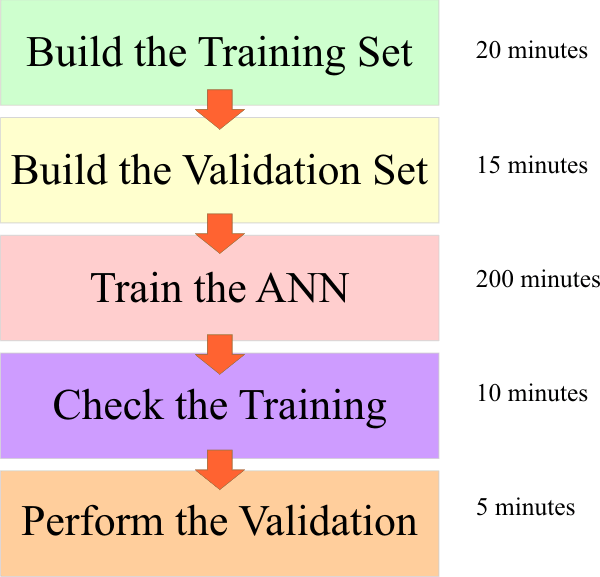# Neurons

## Neuron

The human brain is composed of neurons. The human brain has approximately 100,000 millions of neurons. Each neuron is connected to approximately other 1000 neurons. Similarly, the basic components of an ANN are the neurons. Each neuron is a processing unit.

 Tip An ANN has a fixed number of inputs and outputs. When a signal (a set of numeric values) is applied to the input of an ANN the information flows from one neuron to another. Some sort of input will activate some neurons, while other kind of input will activate other neurons. In the figure below, the sound of the word YES will fully activate the first output neuron, while the sound of the word NO will activate the second neuron at the output of the network.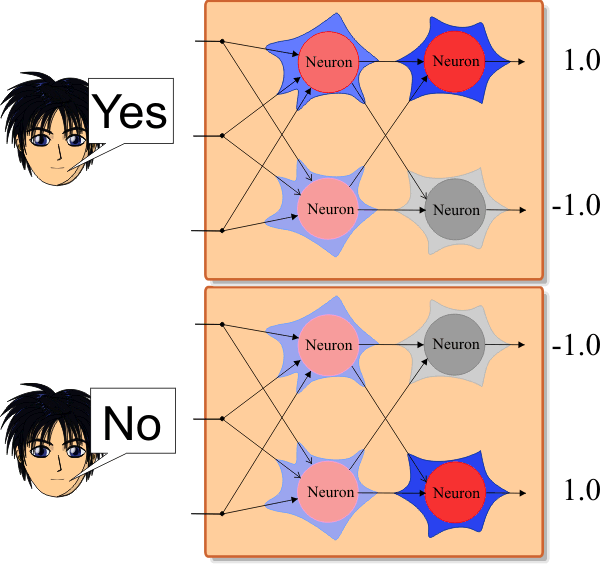## Network Layer

Neurons are arranged in layers inside the ANN. Each layer has a fixed number of neurons. For instance, the ANN shown below has three layers: the input layer, the hidden layer, and the output layer. The hidden layer has three neurons while the output layer has only one neuron.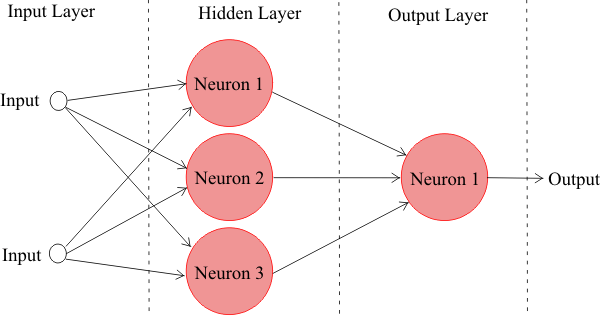Problem 1 Indicate whether the next statement is true or false. The number of outputs in an ANN is always equal to the number of neurons in the output layer.

## Weights

In an ANN, each neuron is connected to another neuron using a weight w which is a numeric value. Each weight has two subscripts. The first subscript indicates the index of the target neuron, while the second subscript represents the index of the source neuron. Because in each layer neurons are numbered using the same indexes, it is very important to indicate which layer the neuron belongs to. Observe that in the figure w11, w12, w13 w21, w22 and w23 are the weights of the output layer. The first three have the same target neuron and hence the first subscript is one. The rest of the weights are connected to the second neuron in the output layer, and the first subscript for these weights is two.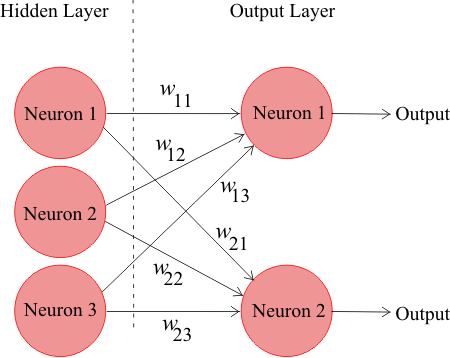Tip Because of the organization of the layer weights, they are commonly represented by a matrix. Thus, each layer has a matrix representing its weights. For example, in the previous figure the output weights are represented by the matrix W.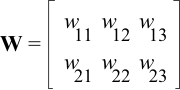Problem 2 Each row in the matrix is associated with the target neuron. What is the meaning of each column in this matrix? What is the meaning of each row in this matrix?

 Problem 3 An ANN has the output weights shown below. Suppose that the input signal is as shown. Compute the values at the input of the neuron located at the output layer.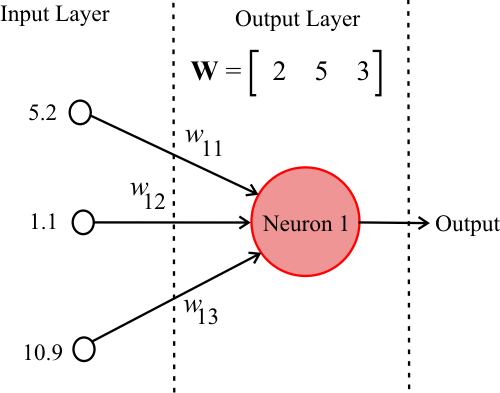Solution 3 Answer: 10.4, 5.5, 32.7

 Tip For practical purposes, an ANN can have zero, one or two hidden layers. Most problems are solved with one hidden layer. Two hidden layers are recommended only for problems that involved discontinuities. An ANN with two hidden layers, two inputs and one output is shown below. The ANN has three neurons in the hidden layer 1, one neuron in the hidden layer 2, and one neuron in the output layer. Typically, the number of inputs and outputs is set by the problem at hand, thus, the network designer has to set appropriately the number of layers and the number of neurons in the hidden layers.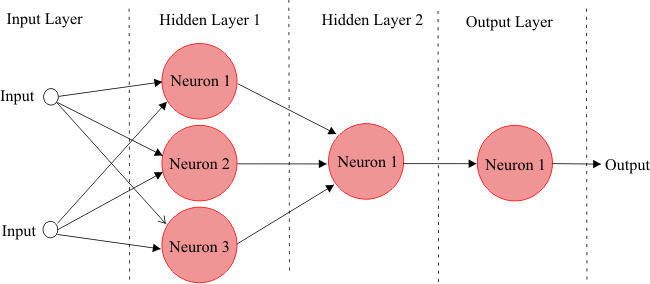Problem 4 An experiment includes the execution of five steps as shown below. The figure shows the steps and their duration in minutes. Each step can be optimized by reducing its duration by 20%. The most important step is the first one (build the training set) as all the other steps depend on this step. A student is authorized to reduce the duration of only one step. What step should the student reduce, if his goal is to optimize the total execution time of the experiment?